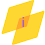Impact-Site-Verification: dbe48ff9-4514-40fe-8cc0-70131430799e

## Search This Blog

MATLAB Program:

% Newton's divided difference
% Find the approximate value of f(1.5) from
% (x,y)= (0,1), (1,e), (2,e^2) & (3,e^3).

n = input('Enter n for (n+1) nodes, n:  ');
x = zeros(1,n+1);
y = zeros(n+1,n+1);

for i = 0:n
fprintf('Enter x(%d) and f(x(%d)) on separate lines:  \n', i, i);
x(i+1) = input(' ');
y(i+1,1) = input(' ');
end
x0 = input('Now enter a point at which to evaluate the polynomial, x = ');

n = size(x,1);
if n == 1
n = size(x,2);
end

for i = 1:n
D(i,1) = y(i);
end

for i = 2:n
for j = 2:i
D(i,j)=(D(i,j-1)-D(i-1,j-1))/(x(i)-x(i-j+1));
end
end

fx0 = D(n,n);
for i = n-1:-1:1
fx0 = fx0*(x0-x(i)) + D(i,i);
end
fprintf('Newtons iterated value: %11.8f \n', fx0)

OUTPUT:

>> Newtons_divided_difference
Enter n for (n+1) nodes, n:  3
Enter x(0) and f(x(0)) on separate lines:
0
1
Enter x(1) and f(x(1)) on separate lines:
1
2.7
Enter x(2) and f(x(2)) on separate lines:
2
7.29
Enter x(3) and f(x(3)) on separate lines:
3
19.683
Now enter a point at which to evaluate the polynomial, x = 1.5
Newtons iterated value:  4.32668750
>>

1.1.